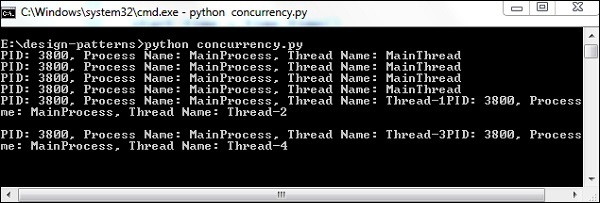# Concurrency in Python

Concurrency is often misunderstood as parallelism. Concurrency implies scheduling independent code to be executed in a systematic manner. This chapter focuses on the execution of concurrency for an operating system using Python.

The following program helps in the execution of concurrency for an operating system −

```import os
import time
import multiprocessing

NUM_WORKERS = 4

def only_sleep():
print("PID: %s, Process Name: %s, Thread Name: %s" % (
os.getpid(),
multiprocessing.current_process().name,
)
time.sleep(1)

def crunch_numbers():
print("PID: %s, Process Name: %s, Thread Name: %s" % (
os.getpid(),
multiprocessing.current_process().name,
)
x = 0
while x < 10000000:
x += 1
for _ in range(NUM_WORKERS):
only_sleep()
end_time = time.time()
print("Serial time=", end_time - start_time)

start_time = time.time()
end_time = time.time()

start_time = time.time()
processes = [multiprocessing.Process(target=only_sleep()) for _ in range(NUM_WORKERS)]
[process.start() for process in processes]
[process.join() for process in processes]
end_time = time.time()

print("Parallel time=", end_time - start_time)
```

### Output

The above program generates the following output −### Explanation

“multiprocessing” is a package similar to the threading module. This package supports local and remote concurrency. Due to this module, programmers get the advantage to use multiple processes on the given system.